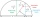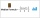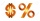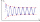# Sequence 11

What is the nth term of this sequence 1, 1/2, 1/3, 1/4, 1/5?

Result

x = (Correct answer is: 1/n)### Step-by-step explanation:

$x=1\mathrm{/}n$Did you find an error or inaccuracy? Feel free to write us. Thank you!Tips to related online calculators
Looking for calculator of harmonic mean?
Looking for a statistical calculator?

## Related math problems and questions:

• HP - harmonic progressionDetermine the 10th term of the harmonic progression 6,4,3,…
• HP - harmonic progressionDetermine the 8th term of the harmonic progression 2, 4/3, 1,…
• Harmonic meanIf x, y, z form a harmonic progression, then y is the harmonic mean of x and z. Find the harmonic mean of the numbers 6 and 5.
• Insert 5Insert five harmonic means between 1/2 and 1/26
• Insert 6Insert four harmonic means between 3/7 and 3/19
• Harmonic seriesInsert four members between 5/3 and 5/11 to form harmonic series (means).
• Sequence 2Write the first 5 members of an arithmetic sequence a11=-14, d=-1
• Harmonic 4The harmonic mean of -6 and 5.
• Median or middleThe number of hours of television watched per day by a sample of 28 people is given below: 4, 1, 5, 5, 2, 5, 4, 4, 2, 3, 6, 8, 3, 5, 2, 0, 3, 5, 9, 4, 5, 2, 1, 3, 4, 7, 2, 9 What is the median value?
• HP - harmonic progression 2Compute the 16th term of the HP if the 6th and 11th term of the harmonic progression are 10 and 18 respectively.
• Sequence 3Write the first 5 members of an arithmetic sequence: a4=-35, a11=-105.
• Missing term 2What is the missing term for the Geometric Progression (GP) 3, 15, 75,__, 1875?
• Percent to interval76% is between which of the following two numbers. 1) 1/5 & 1/4. 2) 1/3 & 1/2 3) 2/3 & 7/10 4) 3/4 & 5/6
• Insert 7Insert five harmonic means between 3 and 18
• Special sequenceWhat if            2×9=1            3×9=2            4×9=3            5×9=4            6×9=5            7×9=6            8×9=7            9×9=8           10×9=9    then 1×9=??? answer with solutions. .. ..
• Five numbers in ratioFive integers are in the ratio 1: 2: 3: 4: 5. Their arithmetic mean is 12. Determine the smallest of these numbers.
• Geometric progression 2There is geometric sequence with a1=5.7 and quotient q=-2.5. Calculate a17.